# Proportions on the GRE

Proportions are extremely common on the GRE. If you don’t have a strong grasp of them, and you are busy trying to figure out combinations/permutations or probability, stop. Focus your attention on mastering proportions before moving on to more tertiary concepts.

So let’s start basic. Proportions can be broken up into two groups: direct proportions and indirect proportions. In this post I am going to focus on direct proportions. They are more intuitive than indirect proportions and are also the more common on the GRE.

## Direct Proportion

Below is an example of a direct proportion: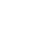To solve for x we cross multiply, giving us: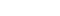;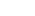.

This is a direct proportion because as the ‘5’ becomes larger (namely it quadruples to become 20) the x also gets larger (it quadruples to become 8). That is both sides are getting larger.

I can almost guarantee that you will not see such a straightforward equation on the GRE Quant section. Instead, you will be given either a word problem or a graph and you will have to translate the information into an equation like the one above.

Let’s take a look at two problems:

In 2004, 2,400 condos sold, 15% of the total housing units sold that year. If 25% of the homes sold in 2004 were four-bedroom houses, then how many four-bedroom homes sold in 2004?

(A)  3,600

(B)  4,000

(C)  4,200

(D) 4,800

(E)  6,000

Explanation

Here we want to set up an equation.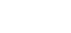;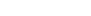;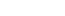. (B).

Some things to note: you can take off the last two zeroes in 2,400 to get 24, a step which will make the math easier. Remember to bring the two zeroes back, which makes sense: x =40, is clearly too low and not amongst the answer choices.

Speaking of answer choices, notice that 25% is less than double of 15%. Therefore, the number of four-bedroom houses sold has to be less than twice the number of condos sold. (D) and (E) cannot be answer. (A) 3600 is only 50% greater than 2,400, so it is probably too low as well. Elimination, esp. if you are short on time, or getting tangled up in the calculation can be very effective.

Now let’s put a spin to this question. Nothing too tricky; indeed you should be able to solve this using the method above.

In 2004, 2,400 condos sold, 15% of the total housing units sold that year. How many units sold in 2004 were not condos?

(A)  16,000

(B)  13,600

(C)  12,400

(D) 11, 200

(E)  8,600

Explanation:

To approach this question, a good idea is to find the number of units in the total market. Then to find the number that are not condos subtract the condos from the total.

My reason for this approach is it is easier to do the math when we are working with 100 vs. 85 (which would be the percent of home that are not condos).

The solution is as follows: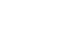;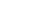. Remember to add the two zeroes: 16,000. Now we have to subtract the total condos (2,400) to find the number of units that are not condos: 16,000 – 2,400 = 13,600.

## Takeaway:

Setting up a proportion is essential to solving a range of GRE math questions. Make sure you can confidently and quickly solve this question type before more on to more challenging – but less common – concepts.